##### Quantum Physics For Dummies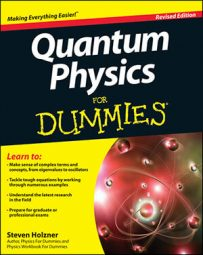One of the major ideas of quantum physics is quantization — measuring quantities in discrete, not continuous, units. The idea of quantized energies arose with one of the earliest challenges to classical physics: the problem of black-body radiation. While Wien’s formula and the Rayleigh-Jeans Law could not explain the spectrum of a black body, Max Planck’s equation solved the problem by assuming that light was discrete.

When you heat an object, it begins to glow. Even before the glow is visible, it’s radiating in the infrared spectrum. The reason it glows is that as you heat it, the electrons on the surface of the material are agitated thermally, and electrons being accelerated and decelerated radiate light.

Physics in the late 19th and early 20th centuries was concerned with the spectrum of light being emitted by black bodies. A black body is a piece of material that radiates corresponding to its temperature — but most ordinary objects you think of as black, such as charcoal, also absorb and reflect light from their surroundings. To make matters easier, physics postulated a black body that reflected nothing and absorbed all the light falling on it (hence the term black body, because the object would appear perfectly black as it absorbed all light falling on it). When you heat a black body, it would radiate, emitting light.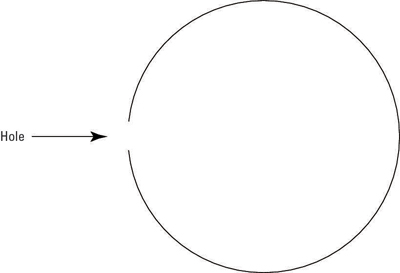A black body.

Well, it was hard to come up with a physical black body — after all, what material absorbs light 100 percent and doesn’t reflect anything? But the physicists were clever about this, and they came up with the hollow cavity you see in the above figure, with a hole in it.

When you shone light on the hole, all that light would go inside, where it would be reflected again and again — until it got absorbed (a negligible amount of light would escape through the hole). And when you heated the hollow cavity, the hole would begin to glow. So there you have it — a pretty good approximation of a black body.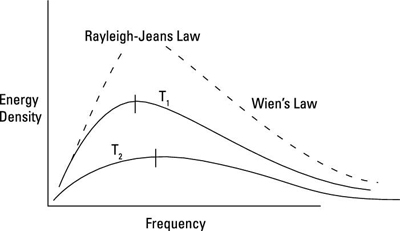You can see the spectrum of a black body (and attempts to model that spectrum) in the above figure, for two different temperatures, T1 and T2. The problem was that nobody was able to come up with a theoretical explanation for the spectrum of light generated by the black body. Everything classical physics could come up with went wrong.

## First attempt: Wien’s Formula

The first one to try to explain the spectrum of a black body was Wilhelm Wien, in 1889. Using classical thermodynamics, he came up with this formula: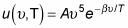In terms of wavelength, it is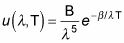They are related by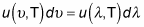Where we have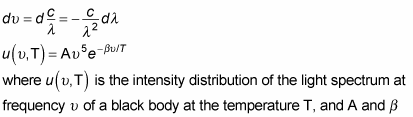are constants which can be measured in experiments. (The spectrum is given by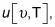which is the energy density of the emitted light as a function of frequency and temperature.)

This equation, Wien’s formula, worked fine for high frequencies, as you can see in the second figure; however, it failed for low frequencies.

## Second attempt: Rayleigh-Jeans Law

Next up in the attempt to explain the black-body spectrum was the Rayleigh-Jeans Law, introduced around 1900. This law predicted that the spectrum of a black body was

The formula should be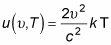In terms of wavelength, the formula is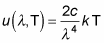where k is Boltzmann’s constant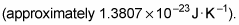However, the Rayleigh-Jeans Law had the opposite problem of Wien’s formula: Although it worked well at low frequencies (see the second figure), it didn’t match the higher-frequency data at all — in fact, it diverged at higher frequencies. This was called the ultraviolet catastrophe because the best predictions available diverged at high frequencies (corresponding to ultraviolet light). It was time for quantum physics to take over.

## An intuitive (quantum) leap: Max Planck’s spectrum

The black-body problem was a tough one to solve, and with it came the beginnings of quantum physics. Max Planck came up with a radical suggestion — what if the amount of energy that a light wave can exchange with matter wasn’t continuous, as postulated by classical physics, but discrete? In other words, Planck postulated that the energy of the light emitted from the walls of the black-body cavity came only in integer multiples like this, where h is a universal constant: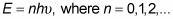With this theory, crazy as it sounded in the early 1900s, Planck converted the continuous integrals used by Raleigh-Jeans to discrete sums over an infinite number of terms. Making that simple change gave Planck the following equation for the spectrum of black-body radiation:

The formula should be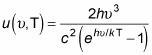This equation got it right — it exactly describes the black-body spectrum, both at low and high (and medium, for that matter) frequencies.

This idea was quite new. What Planck was saying was that the energy of the radiating oscillators in the black body couldn’t take on just any level of energy, as classical physics allows; it could take on only specific, quantized energies. In fact, Planck hypothesized that that was true for any oscillator — that its energy was an integer multiple of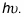And so Planck’s equation came to be known as Planck’s quantization rule, and h became Planck’s constant: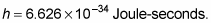Saying that the energy of all oscillators was quantized was the birth of quantum physics.